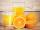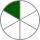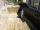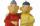# Fraction calculator

The calculator performs basic and advanced operations with fractions, expressions with fractions combined with integers, decimals, and mixed numbers. It also shows detailed step-by-step information about the fraction calculation procedure. Solve problems with two, three, or more fractions and numbers in one expression.

## Result:

### 1/4 + 3/8 = 5/8 = 0.625

Spelled result in words is five eighths.

### How do you solve fractions step by step?

1. Add: 1/4 + 3/8 = 1 · 2/4 · 2 + 3/8 = 2/8 + 3/8 = 2 + 3/8 = 5/8
For adding, subtracting, and comparing fractions, it is suitable to adjust both fractions to a common (equal, identical) denominator. The common denominator you can calculate as the least common multiple of both denominators - LCM(4, 8) = 8. In practice, it is enough to find the common denominator (not necessarily the lowest) by multiplying the denominators: 4 × 8 = 32. In the following intermediate step, the fraction result cannot be further simplified by canceling.
In other words - one quarter plus three eighths = five eighths.

#### Rules for expressions with fractions:

Fractions - use the slash “/” between the numerator and denominator, i.e., for five-hundredths, enter 5/100. If you are using mixed numbers, be sure to leave a single space between the whole and fraction part.
The slash separates the numerator (number above a fraction line) and denominator (number below).

Mixed numerals (mixed fractions or mixed numbers) write as non-zero integer separated by one space and fraction i.e., 1 2/3 (having the same sign). An example of a negative mixed fraction: -5 1/2.
Because slash is both signs for fraction line and division, we recommended use colon (:) as the operator of division fractions i.e., 1/2 : 3.

Decimals (decimal numbers) enter with a decimal point . and they are automatically converted to fractions - i.e. 1.45.

The colon : and slash / is the symbol of division. Can be used to divide mixed numbers 1 2/3 : 4 3/8 or can be used for write complex fractions i.e. 1/2 : 1/3.
An asterisk * or × is the symbol for multiplication.
Plus + is addition, minus sign - is subtraction and ()[] is mathematical parentheses.
The exponentiation/power symbol is ^ - for example: (7/8-4/5)^2 = (7/8-4/5)2

#### Examples:

subtracting fractions: 2/3 - 1/2
multiplying fractions: 7/8 * 3/9
dividing Fractions: 1/2 : 3/4
exponentiation of fraction: 3/5^3
fractional exponents: 16 ^ 1/2
adding fractions and mixed numbers: 8/5 + 6 2/7
dividing integer and fraction: 5 ÷ 1/2
complex fractions: 5/8 : 2 2/3
decimal to fraction: 0.625
Fraction to Decimal: 1/4
Fraction to Percent: 1/8 %
comparing fractions: 1/4 2/3
multiplying a fraction by a whole number: 6 * 3/4
square root of a fraction: sqrt(1/16)
reducing or simplifying the fraction (simplification) - dividing the numerator and denominator of a fraction by the same non-zero number - equivalent fraction: 4/22
expression with brackets: 1/3 * (1/2 - 3 3/8)
compound fraction: 3/4 of 5/7
fractions multiple: 2/3 of 3/5
divide to find the quotient: 3/5 ÷ 2/3

The calculator follows well-known rules for order of operations. The most common mnemonics for remembering this order of operations are:
PEMDAS - Parentheses, Exponents, Multiplication, Division, Addition, Subtraction.
BEDMAS - Brackets, Exponents, Division, Multiplication, Addition, Subtraction
BODMAS - Brackets, Of or Order, Division, Multiplication, Addition, Subtraction.
GEMDAS - Grouping Symbols - brackets (){}, Exponents, Multiplication, Division, Addition, Subtraction.
Be careful, always do multiplication and division before addition and subtraction. Some operators (+ and -) and (* and /) has the same priority and then must evaluate from left to right.

## Fractions in word problems:

• Unknown numberI think the number - its sixth is 3 smaller than its third.
• Cupcakes 2Susi has 25 cupcakes. She gives 4/5. How much does she have left?Mom bake cookies. Rolo took 2/9 of all cookies, Michal 3/9. How many cookies ate Rolo if Michal had 9.
• PC disksPeter has 45 disks in three colors. One-fifth of the disks are blue, red are twice more than the white. How much is blue, red and white disks?
• TripOn the trip drank 3/10 of pupils tea, 2/5 cola, 1/4 mineral water and remaining 3 juice. How many students were on the trip?
• A manA man spends 5/9 of his money on rent, and 5/16 of the remainder on electricity. If the final balance remaining is 550 find how much was spent on rent
• Two pumps togetherThe first pump will fill the tank itself in 3 hours and the second one in 6 hours. How many hours will the tank be full if both pumps are worked at the same time?
• One sixthHow many sixths are two thirds?
• ExcavationExcavation for the base of the cottage has dimensions 4.5 m x 3.24 m x 60 cm. The excavated soil will increase its volume by one-quarter. Calculate the volume of excavated soil.
• DayWhat part of the day are 23 hours 22 minutes? Express as a decimal number.
• CandiesThere are red, blue and green candies in bad. Red to green is in 6:11 ratio and blue to red in a 7: 5 ratio. In what proportion are blue to green candies?
• SummerjobAlbert, Beny, Cyril and Dan worked on summerjob. From the total revenue Albert received 2/5, Beny 1/6 , 3/10 Cyril and Dan the rest which is 324 euros. How many euros earned every one of them?
• University bubbleYou'll notice that the college is up slowly every other high school. In Slovakia/Czech Republic, a lot of people are studying political science, mass media communication, social work, many sorts of management MBA. Calculate how many times more earns cleve